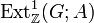# Second cohomology group for trivial group action of locally cyclic torsion-free groups

The goal of this page is to discuss the second cohomology group for trivial group action$H^2(G;A)$ where both$G$ and$A$ are locally cyclic aperiodic groups.

## Computation of the group

We use the formula for second cohomology group for trivial group action of abelian group in terms of Schur multiplier and abelianization (which is a special case of the formula for second cohomology group for trivial group action in terms of Schur multiplier and abelianization), namely the following short exact sequence:$0 \to \operatorname{Ext}^1_{\mathbb{Z}}(G,A) \to H^2(G;A) \stackrel{\operatorname{Skew}}{\to} \operatorname{Hom}(\bigwedge^2G,A) \to 0$

where$\bigwedge^2G = M(G)$ is the exterior square of$G$ and also coincides with the Schur multiplier of$G$.

Note that since locally cyclic implies epabelian, the Schur multiplier of$G$ is the trivial group, and all extensions are themselves abelian groups. In particular,$\bigwedge^2G = 0$, and we get:$H^2(G;A) \cong \operatorname{Ext}^1_{\mathbb{Z}}(G,A)$

Thus, the computation of$H^2(G;A)$ is equivalent to the computation of$\operatorname{Ext}^1_{\mathbb{Z}}(G;A)$.